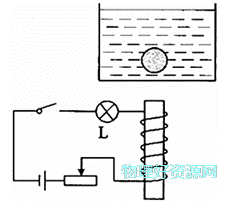# 如图所示，将质量为80g，体积为100cm3的铁制小球放入盛水的薄玻璃容器I/A 0.1 0.2 0.3 0.4 0.5 F/N 0.05 0.1 0.15 0.2 0.25

1）断开开关铁制小球静止时露出水面的体积是多少？

2）闭合开关，调节滑动变阻器的滑片使铁制小球能悬停在水中，此时小灯泡消耗的功率是多大？

3）闭合开关，调节滑动变阻器的滑片使小灯泡正常发光，当铁制小球静止时，小球对容器底的压力是多大？

1条回答
• 解析：

1）小球的密度：

ρ=m/V=80g/100cm3=0.8g/cm3

因小球的密度小于水的密度，所以，断开开关时，铁制小球静止时处于漂浮状态，受到的浮力和自身的重力相等，

则小球受到的浮力：

F=G=mg=80×103kg×10N/kg=0.8N

F=ρgV可得，小球排开水的体积：

V=F/(ρg)=0.8N/(1.0×103kg/m3)

=8×105m3

=80cm3

则小球露出水面的体积：

V=VV=100cm380cm3=20cm3

2）闭合开关，调节滑动变阻器的滑片使铁制小球能悬停在水中时，

因物体浸没时排开液体的体积和自身的体积相等，

所以，小球受到的浮力：

F′=ρgV

=1.0×103kg/m3×10N/kg×100×106m3

=1N

因小球悬停静止时，受到竖直向上的浮力和竖直向下的重力、螺线管对铁制小球的作用力处于平衡状态，

由平衡条件可得：

G+F=F

则螺线管对铁制小球的作用力：

F=FG=1N0.8N=0.2N

查表可知，此时电路中的电流I=0.4A

灯泡的电阻：

RL=UL2/PL=(6V)2/3W=12Ω

此时小灯泡消耗的功率：

PL′=I2RL=0.4A2×12Ω=1.92W

3）当小灯泡正常发光时，电路中的电流：

I=IL=PL/UL=0.5A

查表可知，此时螺线管对铁制小球的作用力

F′=0.25N

此时小球受到竖直向上的浮力和支持力、竖直向下的重力和螺线管对铁制小球的作用力处于平衡状态，

由力的平衡条件可得：

F+F支持=F′+G

则小球受到的支持力：

F支持=F′+GF′=0.25N+0.8N1N=0.05N

因小球对容器底的压力和受到的支持力是一对相互作用力，

所以，小球对容器底的压力：

F=F支持=0.05N

讨论(1)看不清?